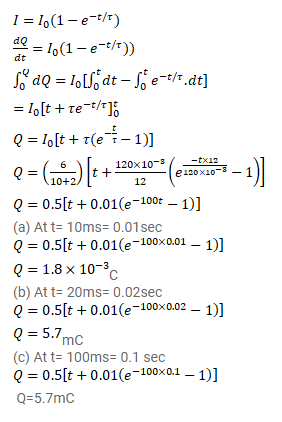# An inductor-coil of resistance 10Ω and inductance 120mH

Question:

An inductor-coil of resistance $10 \Omega$ and inductance $120 \mathrm{mH}$ is connected across a battery of emf $6 \mathrm{~V}$ and internal resistance $2 \Omega$. Find the charge which flows through the inductor in

(a) 10ms,

(b) $20 \mathrm{~ms}$ and

(c) $100 \mathrm{~ms}$ after the connections are made.

Solution: